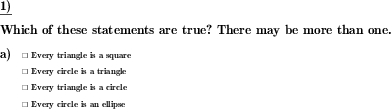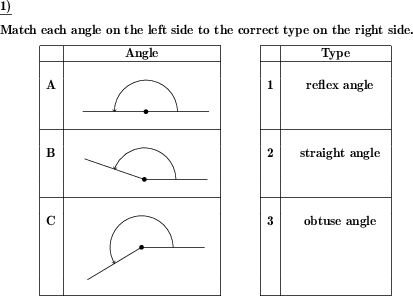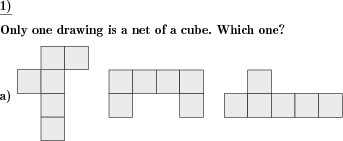Custom math worksheets at your fingertips# Details for problem "A square is also a rectangle"

Quickname: 9445

Elementary School, Primary School, Junior High School, Middle School.

## Summary

Identify correct statements for 2d geometric shapes

## Example## Description

A series of statements is made in the form of "Every square is a rectangle" or "Every triangle is a circle". For every statement, it has to be determined whether it is true or not.

Possible shapes are:

- rectangle
- square
- parallelogram
- rhombus
- trapezoid

Other shapes:

- circle
- ellipse
- triangle

For every shape a selection can be made whether it may occur.

For the question, it can be chosen that only one statement in every series of statements will be true, or that more than one may be true. In the latter case, the number of correct statements can be provided or not. This is selectable.

The number of problems is selectable, too.

Download free printable worksheets for this math problem here. The worksheet contains the problems only, the solution sheet includes the answers. Just click on the respective link.

•Worksheet 1Solution sheet with answers
•Worksheet 2Solution sheet with answers
•Worksheet 3Solution sheet with answers

If you can not see the solution sheets for download, they may be filtered out by an ad blocker that you may have installed. If this is the case, please allow ads for this page and reload the page. The solution sheets will then reappear.

• Do these sample worksheets do not really fit?
• Do you need more math worksheets, with a different level of difficulty?
• Would you like to combine different problems on a worksheet and adjust them to your needs?
• As a teacher, you can put together your own worksheets using the automatically generated math problems provided.
With a free initial credit, you can start creating your own math worksheets in a few minutes.

You can try it for free! Register here, to create custom worksheets now!

## Customization options for this problem

Parameter
Possible values
Number of problems
1, 2, 3, 4, 5, 6
Rectangles
no, rectangle, square, square,rectangle
Triangle
Yes, No
Circle,Ellipse
no, ellipse, circle, circle,ellipse
Parallelogram,Rhombus
no, parallelogram, rhombus, parallelogram,rhombus
Trapezoid
Yes, No
Question

## Similar problems

Remark
Description
Identify two-dimensional geometric shape
Name the two dimensional flat geometric shape shown

## Other types of problems that appear on worksheets with this problem:

Relevance
Name
Description
Quickname
Example
****
Angle types
For a given angle, the correct type has to be marked.****
Match angles to angle types
Given angles have to be matched to given types****
Identify cube nets
A series of drawings is shown. Which ones represent cube nets?****
Area of a triangle from side and height
The lengths of a side of a triangle and the corrensponding height are given. Calculate the area of the triangle.Deutsche Version dieser Aufgabe
These informational pages with samples describe math problems that can be combined on custom math worksheets with solutions for home and K-12 school use.
Deutsche Seiten
×